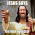## Tuesday, November 11, 2014

### NFL Picks Contest- Week 10 Results and Standings

Here are the Week 10 results and standings in the NFL Picks Contest. OneAndDone stays on top, but MOJO is nipping at his heels. Cokeboy had a huge week thanks to Aaron Rodgers' right arm.

Here are the current standings;

OneAndDone- 27+17+17+34+41+5+31+38+16+24 = 250 pts.
MOJO-  27+17+17+29+16 +24+32+37+16+34 = 249 pts.
lightning36- 27+17+17+29+16+24+31+24+20+34 = 239 pts.
Captain Crunch- 27+17+17+29+16+5+35+38+24+24 = 232 pts.
cokeboy99- 27-(8)+21+34+16+13+20+38+16+51 = 228 pts.
mrben09- 27+17+17+29+16+24+31+37-3+24 = 219 pts.

ggrouchie- 27+17+17+34+41+13+20-(3)+24+24 = 214 pts.
TwoMinuteWarning- (3)+17+37+29+16-(7)+35+37+20+24 = 205 pts.
Vegas Vic- 27+17+21+29+16+5+32-(3)+20+31 = 195 pts.
Music City Momma- 13+17+21+29+16-(7)+32+38-(3)+31 = 187 pts.

Coach- 27+17+21+29+20+24+20-(3)+16-(7) = 164 pts.
M Prosk- (3)+ 0+ 0+34+41+13+11+20+20+24 = 160 pts.
Neophyte- 27+17-(19)+0+41+24+21+0+24+24 = 159 pts.
Grange95- (3)+17+21+29+16+24+11+20+16+0 = 151 pts.
TeamMiRketti- 27+17+17-(3)+16+24+0-(19)+20+34 = 133 pts.

Week 10 scores

angerisagift- Baltimore (W 21-7) = 24 pts.
MOJO- Denver (W 41-17) = 34 pts.
Vegas Vic- Seattle (W 38-17) = 31 pts.
Captain Crunch- Baltimore (W 21-7) = 24 pts.
OneAndDone- Baltimore (W 21-7) = 24 pts.
mrben09- Baltimore (W 21-7) = 24 pts.

ggrouchie- Baltimore (W 21-7) = 24 pts.
Coach- Pittsburgh (L 13-20) = (7 pts.)
TeamMiRketti-  Denver (W 41-17) = 34 pts.
lightning36- Denver (W 41-17) = 34 pts.
Neophyte- Baltimore (W 21-7) = 24 pts.

Music City Momma- Seattle (W 38-17) = 31 pts.
TwoMinuteWarning- Baltimore (W 21-7) = 24 pts.
Grange95- 0 pts.
M Prosk- Baltimore (W 21-7) = 24 pts.
cokeboy99- Green Bay (W 55-14) = 51 pts.

Thanks for playing.

Week 11 picks are due by Saturday at 6pm (CDT). Only Sunday and Monday games are eligible.

Good luck!

#### 1 comment:

1.the sheeeeeeeeeeit is getting real LOL